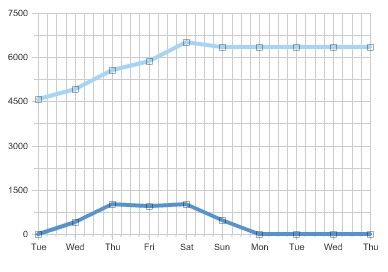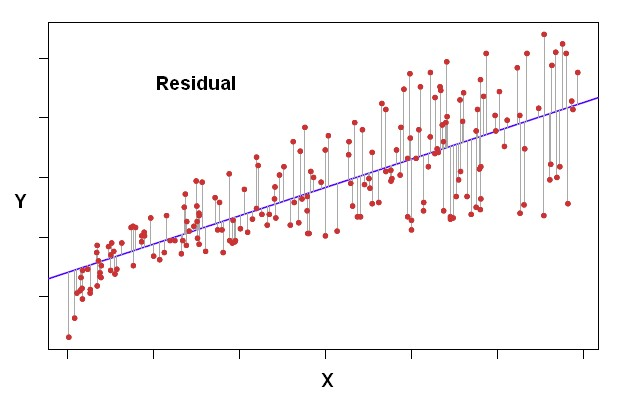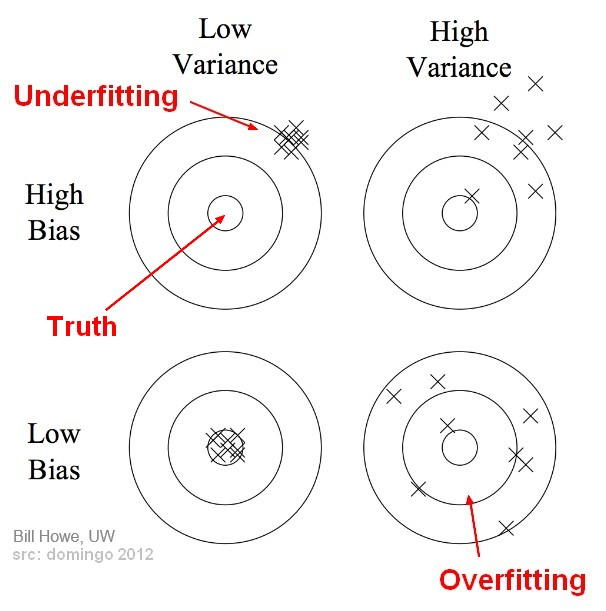# Statistics - Deviation Score (for one observation)

The deviation score is the difference between two scores as :

• a value $X$
• and an other value (usually the predicted score: mean, …)

## Example

• the mean $\bar{X}$ of a distribution for the calculation of the correlation coefficient
• or a predicted variable for the calculation of a regression coefficient

## Deviation Form

By putting all scores of a variable in a deviation form, the average for this variable will be equal to zero. It's called centering.

To center means to put in deviation form.

Discover MoreData Visualization - Line Chart

Line chart shows quantities: over time. time series or by category. Line graphs are useful for showing: trends over time. deviations between values. Can be used to plot multiple (variables|measures)...Distance

is a numerical description of how far apart objects are. Same as: In most cases, “distance from A to B” is interchangeable with “distance between B and A”. In physics or everyday...Alerting is the science of detection Deviations from prediction on Time serie are a powerful way to tell when there is a problem and to trigger alerts when a is reached. See also: ...Statistics - (Residual|Error Term|Prediction error|Deviation) (e| )

The residual is a deviation score measure of prediction error in case of regression. The difference between an observed target and a predicted target in a regression analysis is known as the residual...Statistics - (Total) Sum of the square (TSS|SS)

SS or TSS is the sum of the square deviation scores from the mean for one variable. It's the error calculation of the no predictor model (The basis model).Statistics - (Variance|Dispersion|Mean Square) (MS)

The variance shows how widespread the individuals are from the average. The variance is how much that the estimate varies around its average. It's a measure of consistency. A very large variance means...Statistics - Centering Continous Predictors

By putting all scores of a variable in a deviation form, the average for this variable will be equal to zero. It's called centering. To center means to put in deviation form. To center a variable, take...Statistics - Correlation Matrix

From a raw matrix to a correlation matrix. A correlation matrix is a special matrix used in statistics. It is a square symmetric matrix. 3 columns (3 variables), 8 rows (8 individuals) ...Statistics - Cross Product (of X and Y) (CP|SP)

The cross product is a calculation used in order to define the correlation coefficient between two variables. SP is the sum of all cross products between two variables. For one observation (one...Statistics - Little r - (Pearson product-moment Correlation coefficient)

The Pearson product-moment correlation coefficient is a correlation coefficient formulas that can be applied when both variables are continuous. The correlation is the standardized Covariance as...